Courses
Courses for Kids
Free study material
Free LIVE classes
More

# Maths Worksheet for Kids- Tetris Math is FunLIVE
Join Vedantu’s FREE Mastercalss

## Maths Worksheet for Kids| Tetris Math is Fun - Download Free PDF with Solutions

Tetris is Math is fun is one of the important chapters in the Maths syllabus for KG-3 students. This topic is fun and interactive as it takes the medium of a fun and interesting game to help children learn basic math skills. Students can study different patterns and build new figures with the help of the Tetris game. The experts at Vedantu have created a worksheet for children to help them understand the concept of mathematics with a game. Download Tetris Math is fun, and answer the questions in the worksheets easily.

The students of KG-3 will benefit from practising these worksheets for Tetris math. Not only will they be able to understand the concept of Tetris, but they will also be able to decipher the meaning of different mathematical terms such as area, perimeter, and more from the game. With the help of these Tetris math games, they can have an interactive learning session.

### Did You Know?

Tetris is one of the most popular video games to be featured in the Guinness Book of World Records as a top-selling game worldwide. Playing the Tetris game has many benefits, as it can help boost children's cognitive and logical abilities.

Last updated date: 28th Sep 2023
Total views: 22.2k
Views today: 1.22k

## Access Worksheet for Maths KG-2 Tetris Worksheet Play With Shapes

Tetris is a puzzle video game. Players in the Tetris game clear lines by rearranging the variously shaped pieces which fall onto the playing field. To continue, the player must fill in the empty spaces after the completed lines vanish and award the player points. The game is over when the lines that haven't been cleared reach the top of the playing field.

The player's score will increase the longer they can postpone this result. In multiplayer games, players must outlive their rivals; in some variations, players can impose penalties on rivals by successfully completing a sizable number of lines. Some versions change the rules by including additional features like three-dimensional displays or a method for reserving pieces.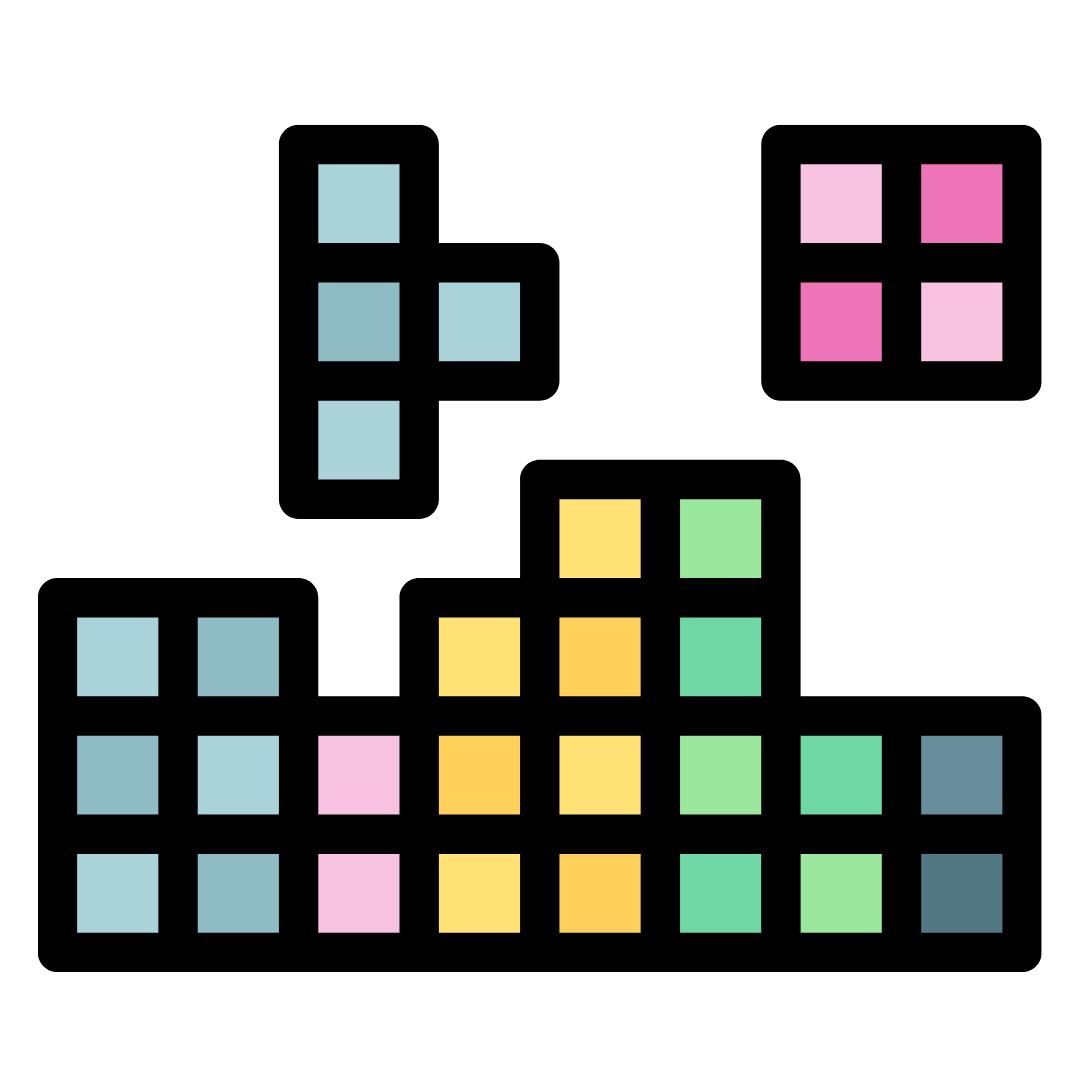Tetris

### QUESTIONS:

1. How many yellow blocks are there?

2. What is the shape of the blue block in the above figure?

3. How many violet Tetris are there?

4. How many green Tetris are there?

5. What is the area covered by a yellow-shaped box?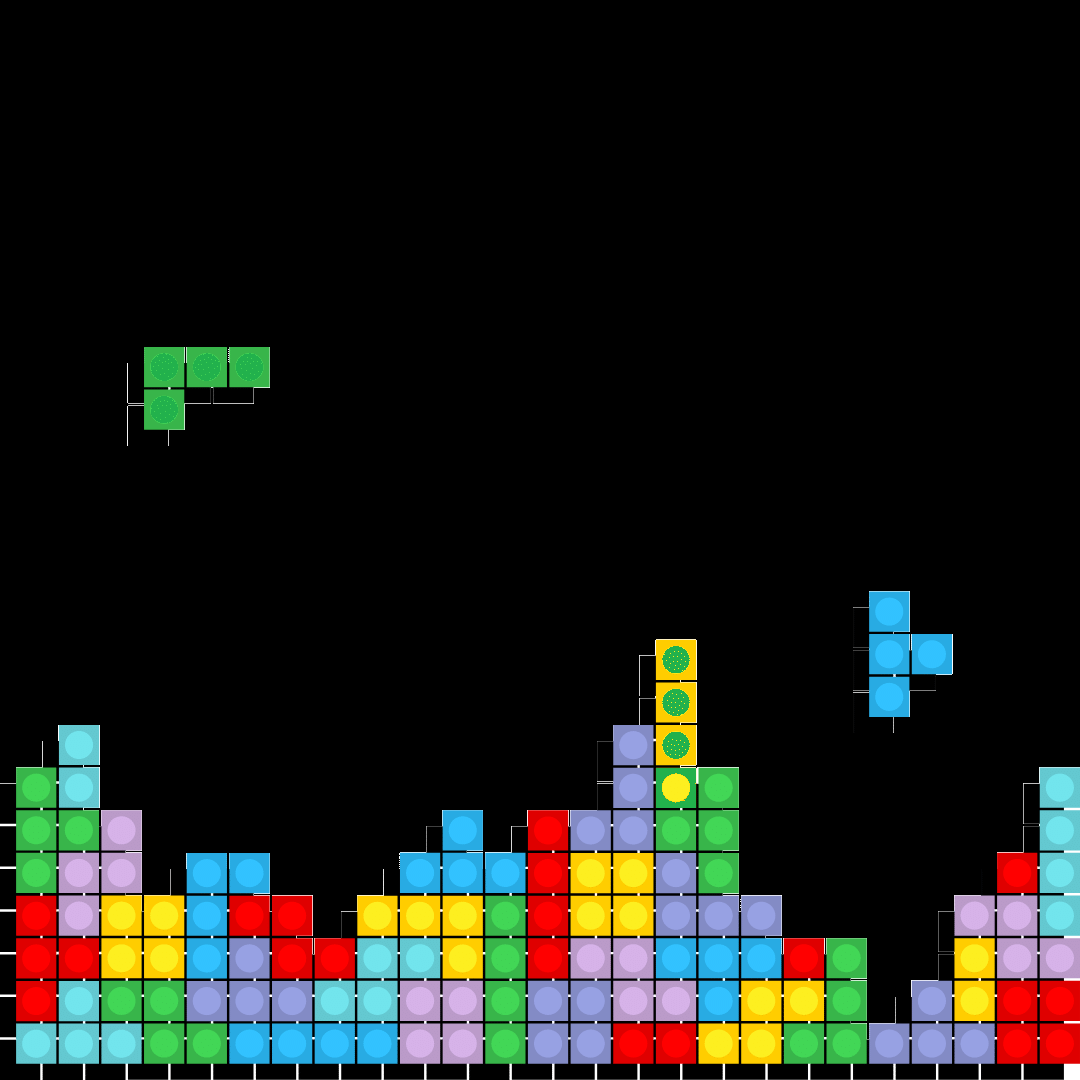Tetris

6. What is the area covered by T shaped block?

7. What is the area covered by the orange block?

8. What is the area covered by the “o” yellow block?

9. How many red blocks are there?

10. How many blue blocks are there?tetris depicting alphabet “T”

11. Which shape does this block suggest?“s” shape block

12. Which shape of the alphabet does this block suggest?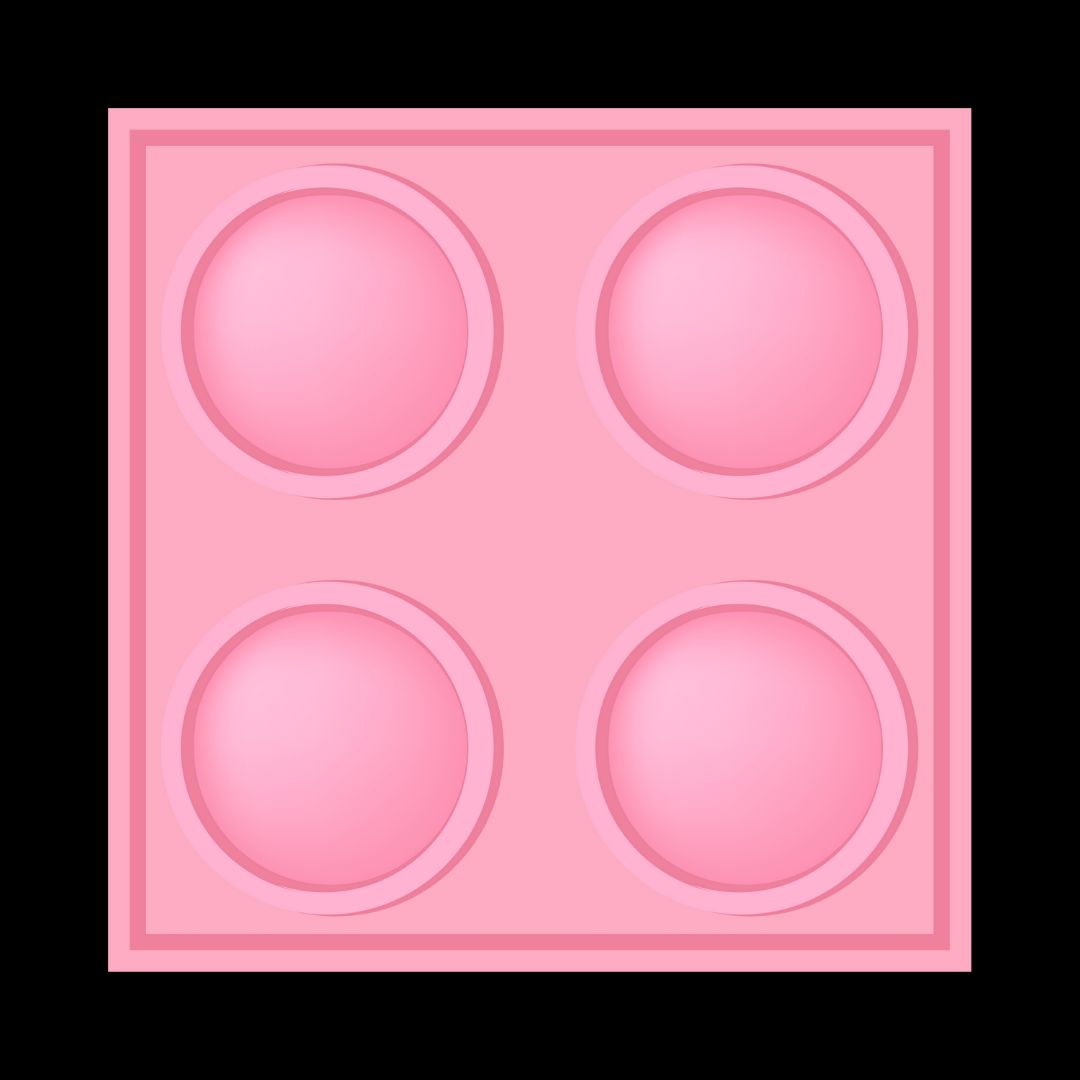“o” yellow block

13. Which shape of the alphabet does this block suggest?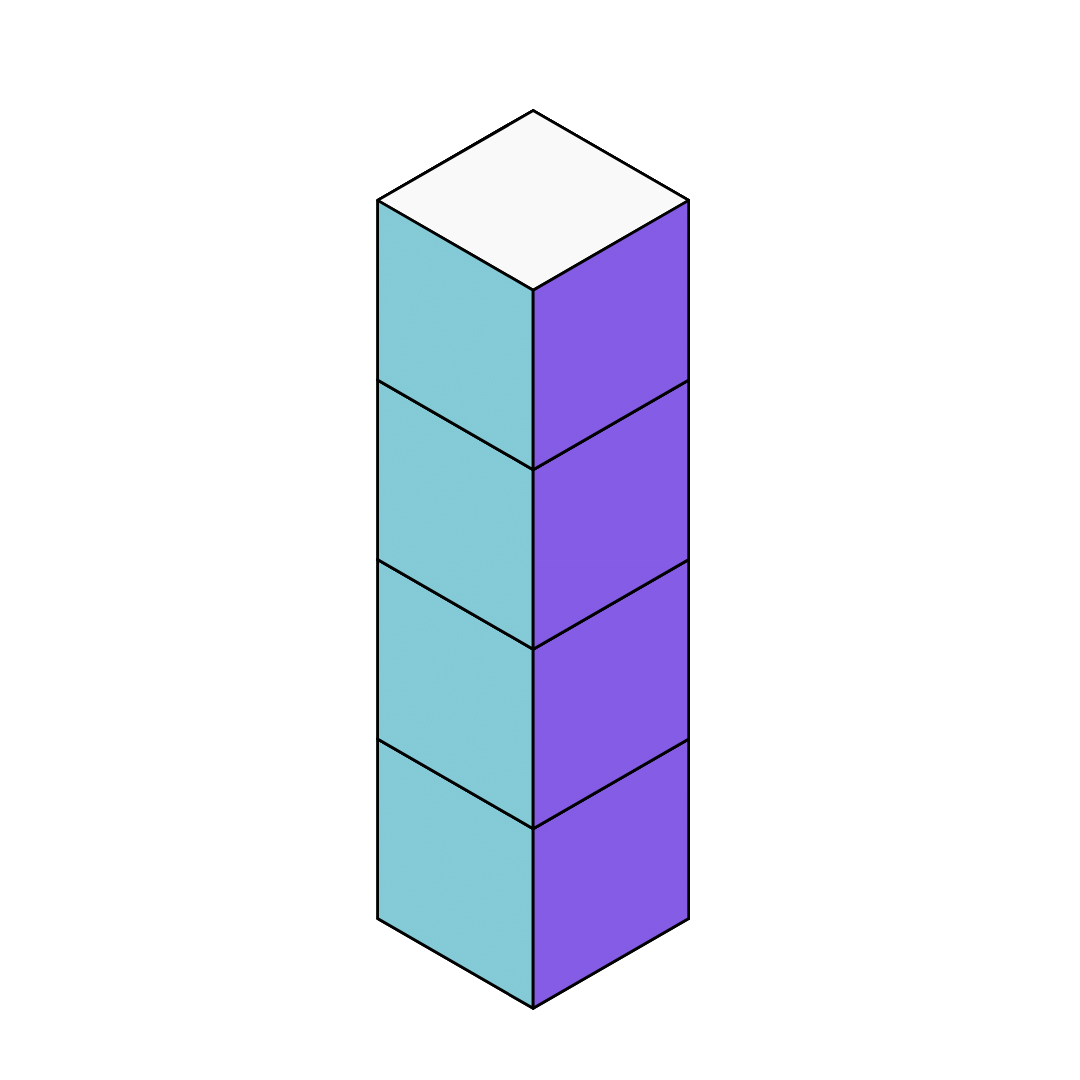“I”  is the shape of the blue block

14. Which shape of the alphabet does this block suggest?

15. What is the shape of the Z block?

1.  Seven different tetrominoes, or geometric figures, are used in this game: an S, Z, T, L, Line, Mirrored L, and a Square. There are four yellow blocks in the Tetris depicted above.

2. “I”  is the shape of the blue block.

3. There are four violet blocks in Tetris.

4. There are four blocks of green Tetris.

5. The area covered by yellow Tetris is 4 units.

6. The area covered by T shaped block is 4 units.

7.  The area covered by the orange block is 8 units.

8. The area covered by the “o” yellow block is 8 units.

9. There are twenty red blocks in the following Tetris.

10. There are sixteen blue blocks in Tetris.

11. we have seven different shapes called tetrominoes: S-shape, Z-shape, T-shape, L-shape, Line-shape, Mirrored L-shape, and square-shape. The block in the image suggests the “T” shape alphabet.

12. The block suggests an “s” shape block with 4 units area.

13. The block suggests an “O” shaped block.

14. A falling brick puzzle game is Tetris. Seven different tetrominoes, or geometric figures, are used in this game: an S, Z, T, L, Line, Mirrored L, and a Square. Four squares are used to create each of these shapes. On the board, the forms are tumbling down. Moving and rotating the shapes will help them fit as tightly as possible in the Tetris game. The block suggests an “I” shaped block.

15. The shape of the Z block is: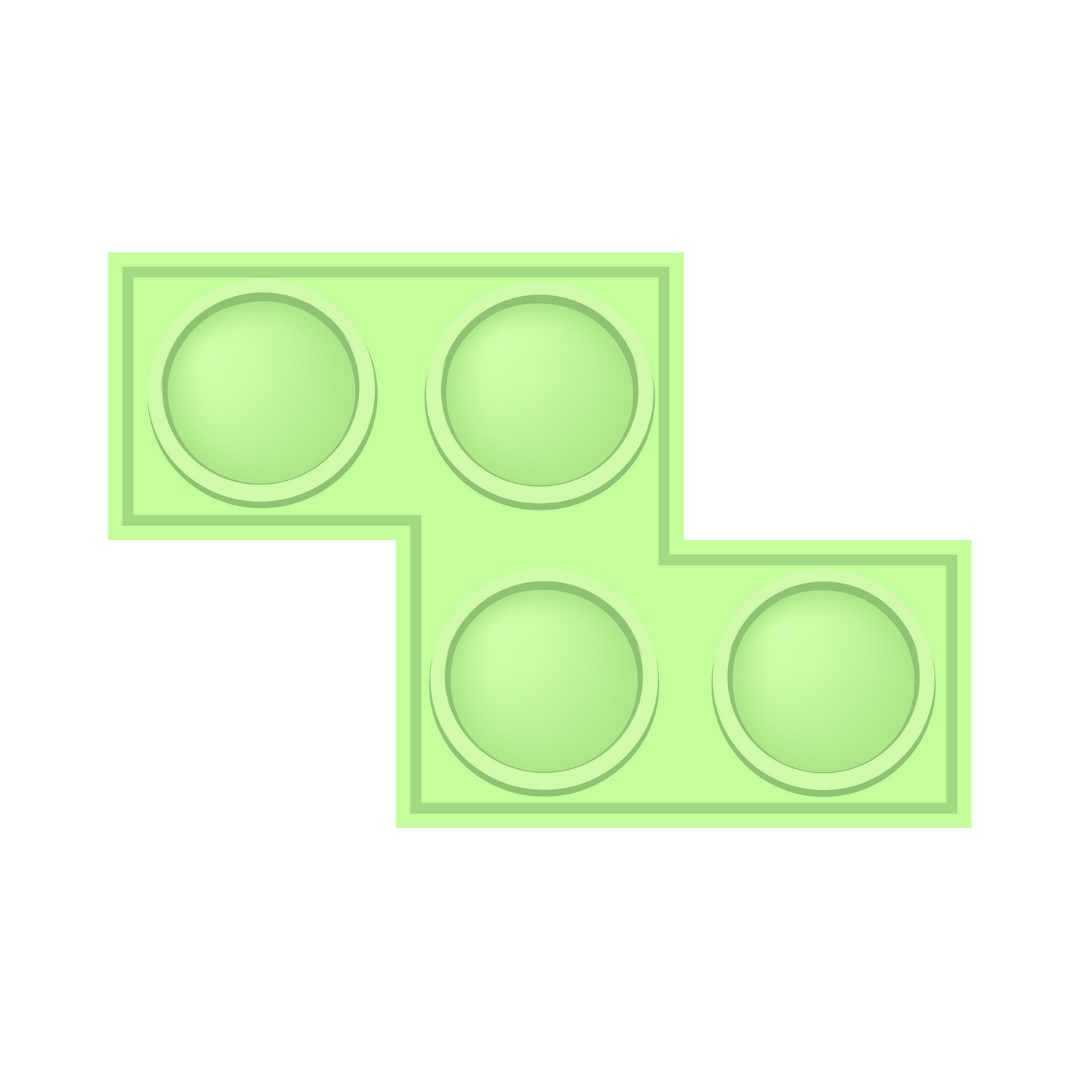Z block

## Importance of Tetris Math is Fun Worksheet for Students

• The chapter on Tetris Math is Fun created a basic foundation for understanding the concept of different shapes and figures using the game. Children will place different shapes and colours together to create new patterns and shapes on their own.

• They will also be asked questions about the areas and perimeters of the shapes to boost their calculative skills as well. Using the game of Tetris as a teaching subject is surely a fascinating idea. That is why this topic is extremely important for KG-3 students.

• The answers and explanations of the questions are also provided in the Tetris Math is Fun Worksheet to help the students understand the concepts better. There are rectilinear shapes, squares, rectangles, and many other units that the students can group together to form new and amazing patterns.

• Students will figure out how different shapes and colours can be matched together to create beautiful designs, thus boosting their creativity.

### Benefits of Practicing Tetris Math is Fun Worksheets

There are a lot of benefits that students of KG-3 will gain from referring to and solving the worksheets. Here are some benefits mentioned below:

• The Tetris game has been explained in a simple way for the students to understand. They can learn about different shapes and figures from the game.

• There are different questions asked about the areas and perimeters of different shapes. Students can solve these questions to improve their mathematical skills.

• Students can boost their creativity by creating new designs and patterns by experimenting with colours and shapes.

• All the questions and activities have been explained by all the experts. Hence, students can understand the question format and hence figure out the answer format as well.

Do you want to improve your concepts in mathematics in a fun and interesting manner? Well, download Tetris Math Games and worksheets from Vedantu to practice more and improve your knowledge. These worksheets have been designed by experts to help your learning process.

## FAQs on Maths Worksheet for Kids- Tetris Math is Fun

1. Where can I find Tetris Math is Fun worksheets?

You can download the Tetris Math is Fun Worksheets from the website of Vedantu. There are different worksheets available for you to boost your practice.

2. How to play the Tetris game?

In the Tetris game, you can place the constantly falling blocks of different shapes on top of one another to create different patterns.

3. What is the cost of the Tetris Math is Fun worksheet?

The Tetris Math is Fun worksheet is available for free at the official website of Vedantu.

4. Can I get better in Maths with Tetris games?

Yes, you can get better in Maths with these games. The worksheets will help you answer mathematical questions about the geometry of the shapes.

5. How is Math used in Tetris?

Tetris is a combination of geometry and calculus. Students will be able to learn the concepts of areas and perimeters from the game.